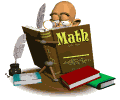• What We Are Learning In Math This QuarterStudents should be able to:

1. Know the place and value of whole numbers through a million and decimal numbers to thousandths (to check this ask your child to create a place value chart)

2. To know how to find volume and explain the formula for volume (length x width x height)

3. Able to accurately add and subtract decimal numbers (decimals and places should be lined up )

4. Able to write numbers in various ways.    example:  375.7,  three hundred seventy-five and seven tenths, 300+70+5+ .5,  (3x100) + (7x10) +(5x1)+(7x .1)

5. Rounding decimals and whole number to a specific place.  ( Children should be beyond the skill and steps of rounding, they should understand how they found their answer and why their answer is correct)